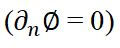top of page
Search
• manchestercfd

# Quick overview of different ‘Boundary Conditions’ in CFD

When solving the Navier-Stokes equation (i.e. the fluid’s governing equations) and continuity equation, appropriate initial conditions and boundary conditions need to be applied. Various types of boundary conditions will be discussed in more detail in this lecture.

Neumann and Dirichlet Boundary Conditions

• When using a Dirichlet boundary condition, one prescribes the value of a variable at the boundary, e.g.• When using a Neumann boundary condition, one prescribes the gradient normal to the boundary of a variable at the boundary, e.g.• Note that at a given boundary, different types of boundary conditions can be used for different variables.

Inlet

Inflow

• Transported variables specified on the boundary, either by a predefined profile or (user’s preference) by doing an initial 1D, fully-developed-flow calculation.

Stagnation (or reservoir)

• Total pressure and total temperature (in compressible flow) or total head (in incompressible flow) fixed; usual inflow condition for compressible flow.

Outlet

Outflow

• Zero normal gradient for all variables.Pressure

• As for outflow, except fixed value of pressure; usual outlet condition in compressible flow if the exit is subsonic.

Symmetry

• Symmetry boundary conditions are those that arise by viewing the computational domain as a sub-region of some larger domain which possesses planes or axes of symmetry.

• In a typical CFD code, plane two-dimensional flows can be simulated using a single layer of cells bounded on its sides by symmetry planes, since there is no velocity component in the spanwise direction.

• Symmetry boundary condition can also be applied to axially symmetric flows, for instance by generating a single segment of cells and applying the symmetry conditions on the segment surfaces.

• Applying symmetry boundary conditions can significantly reduce the mesh size and consequently results in considerable savings in computational time.

Periodic (Cyclic)

• This type of boundary condition consists of pairs of geometrically identical boundaries at which all flow conditions are matched.

• A simple example is that of a 2D channel with both walls roughened by a repeating obstacle.

• By using symmetry and cyclic boundary conditions, it is possible to solve only a small section made up of one symmetry plane and one pair of cyclic boundary conditions.

• In applying periodic boundary conditions, special treatment of the transport equations is required: by adding extra source terms to the momentum and energy equations, the streamwise pressure drop and temperature increase due to heating are accounted for.Rotational Periodicity

• ΔP = 0 across periodic planes.

• Axis of rotation must be defined in fluid zone.

• Translational periodicity.

• ΔP can be finite across periodic planes.

• Models fully developed conditions.

• Specify either mean ΔP per period or net mass flow rate.Wall Boundary Conditions

• Wall boundaries can be defined as no-slip or slip walls

• In viscous flows, no-slip conditions are applied at walls.

• The wall thermal boundary conditions are usually defined as constant temperature or constant heat flux.

• Special treatments are available to represent wall roughness.

## Recent Posts

See All

1. Modes of Heat Transfer Conduction, convection and radiation are the three mechanisms by which thermal energy may be transferred from one point in space (and time) to another. • Conduction: diffusio

bottom of page